Students can Download Tamil Nadu 11th Chemistry Model Question Paper 1 English Medium Pdf, Tamil Nadu 11th Chemistry Model Question Papers helps you to revise the complete Tamilnadu State Board New Syllabus and score more marks in your examinations.

## TN State Board 11th Chemistry Model Question Paper 3 English MediumTime: 2½ Hours
Total Score: 90 Marks

General Instructions:

1. The question paper comprises of four parts.
2. You are to attempt all the parts. An internal choice of questions is provided wherever applicable.
3. All questions of Part I. II, III and IV are to be attempted separately.
4. Question numbers 1 to 15 in Part I are Multiple Choice Questions of one mark each. These are to be answered by choosing the most suitable answer from the given four alternatives and writing the option code and the corresponding answer.
5. Question numbers 16 to 24 in Part II are two-mark questions. These are to be answered in about one or two sentences.
6. Question numbers 25 to 33 in Part III are three-mark questions. These are to be answered in about three to five short sentences.
7. Question numbers 34 to 38 in Part IV are five-mark questions. These are to be answered in detail. Draw diagrams wherever necessary.

PART -1

Choose the most suitable answer from the given four alternatives.

Question 1.
The equivalent mass of a trivalent metal element is 9 g eq-1 the molar mass of its anhydrous oxide is ………………………… .
(a) 102 g
(b) 27 g
(c) 270 g
(d) 78 g
(a) 102 g

Let the trivalent metal be M3+
Equivalent mass = mass of the metal / valance factor
9 g eq-1 = mass of the metal/3 eq
Mass of the metal = 27g Oxide formed M2O3;
Mass of the oxide = (2 x 27) + (3 x 16) = 102gQuestion 2.
Based on equation E = – 2,178 x 10-18 J $$\left(\frac{z^{2}}{n^{2}}\right)$$ certain conclusions are written.
Which of them is not correct? [NEET]
(а) Equation can be used to calculate the change in energy when the electron changes orbit
(b) For n = 1, the electron has a more negative energy than it does for n = 6 which means that the electron is more loosely bound in the smallest allowed orbit.
(c) The negative sign in equation simply means that the energy of electron bound to the nucleus is lower than it would be if the electrons Were at the infinite distance from the nucleus.
(d) Larger the value of n, the larger is the orbit radius.
(b) For n = 1, the electron has a more negative energy than it does for n = 6 which means that the electron is more loosely bound in the smallest allowed orbit.

Correct statement: For n = 1, the electron has more negative energy than it does for n = 6 which means that the electron is strongly bound in the smallest allowed orbit.

Question 3.
Match the following.
List-I – List-II
A. Jewels – 1. Sodium chloride
B. Bolts and cot – 2. Copper
C. Table salt – 3. Gold
D. Utensils – 4. Iron
Code: A B C D
(a) 3 4 1 2
(b) 4 1 3 2
(c) 1 4 2 3
(d) 2 3 4 1
(a) 3 4 1 2Question 4.
The reaction H3PO2 + D2O → H2DPO2 + HDO indicates that hypo-phosphorus acid is …………… .
(a) tribasic acid
(b) dibasic acid
(c) mono basic acid
(d) none of these
(c) mono basic acid

Hypophosphorous acid on reaction with D2O, only one hydrogen is replaced by deuterium and hence it is mono basic.

Question 5.
RbO2 is …………….. .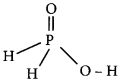(a) superoxide and paramagnetic
(b) peroxide and diamagnetic
(c) superoxide and diamagnetic
(d) peroxide and paramagnetic
(a) superoxide and paramagneticQuestion 6.
At identical temperature and pressure, the rate of diffusion of hydrogen gas is 3 $$\sqrt{3}$$ times that of a hydrocarbon having molecular formula Cn H2n-2. What is the value of n?
(a) 8
(b) 4
(c) n3
(d) 1
(b) 4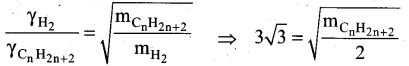Squaring on both sides and rearranging
27 x 2 = mCnH2n-2
54 = n(12) + (2n-2) (1)
54 = 12n + 2n – 2 = 14n – 2
n = (54 + 2)/14 = 56/14 = 4

Question 7.
Statement-I: All living things are open systems.
Statement-II: Because they continuously exchange matter and energy with the surroundings,
(a) Statement-I and II are correct and Statement-II is the correct explanation of Statement-I.
(b) Statement-I and II are correct but Statement-II is not the correct explanation of Statement-I.
(c) Statement-I is correct and Statement-II is wrong.
(d) Statement-I is wrong but Statement-II is correct.
(a) Statement-I and II are correct and Statement-II is the correct explanation of Statement-I.Question 8.
The values of Kp1 and Kp2 for the reactions: $$\mathrm{X} \rightleftharpoons \mathrm{Y}+\mathrm{Z} \text { and } \mathrm{A} \rightleftharpoons 2 \mathrm{B}$$ are in the ratio 9 : 1 if degree of dissociation and initial concentration of X and Abe equal then total pressure at equilibrium P1 and P2 are in the ratio ………………………… .
(a) 36: 1
(b) 1: 1
(c) 3: 1
(d) 1: 9
(a) 36: 1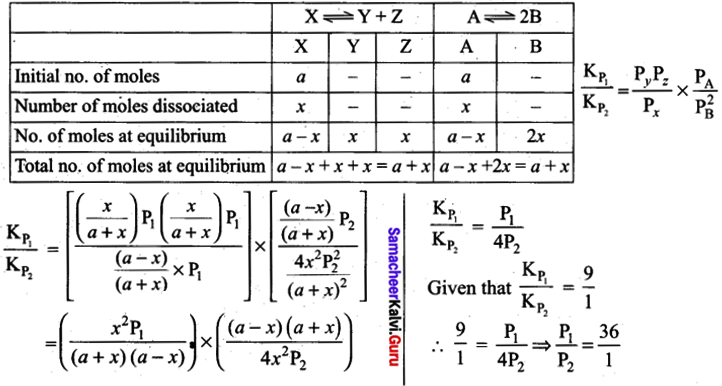Question 9.
Which of the following is not an non-ideal solution showing negative deviation?
(a) Phenol and aniline
(b) Ethanol and water
(c) Acetone + Chloroform
(d) n-Heptane and n-Hexane
(d) n-Heptane and n-HexaneQuestion 10.
In C1F3, NF3 and BF3 molecules the chlorine, nitrogen and boron atoms are …………………. .
(a) sp3 hybridised
(b) sp3, sp3 and sp2 respectively
(c) sp2 hybridised
(d) sp3d, sp3 and sp2 hybridised respectively
(d) sp3d, sp3 and sp2 hybridised respectively

Solution: C1F3 – sp3d hybridisation ; NF3 – sp3 hybridisation ; BF3 – sp2 hybridisation

Question 11.
The number of stereoisomers of 1, 2-dihydroxy cyclopentane is
(a) 1
(b) 2
(c) 3
(d) 4
(c) 3

Question 12.
Consider the following statement.
(i) Covalent bonds .are break into two different ways.
(ii) They are Homolytic and Heterolytic cleavage.
(iii) During the fission of a covalent bond, organic compounds form a new organic molecules. Which of the above statement is/are not correct?
(a) (i) only
(b) (i) and (ii)
(c) (iii) only
(d) (ii) and (iii)
(c) (iii) onlyQuestion 13.
Major product of the below mentioned reaction is ………………….. .
$$\left(\mathrm{CH}_{3}\right)_{2} \mathrm{C}=\mathrm{CH}_{2} \stackrel{\mathrm{ICl}}{\longrightarrow}$$
(a) 2-chloro -1- iodo – 2 – methylpropane
(b) l-chloro-2-iodo-2-methylpropane
(c) 1,2 – dichloro – 2 – methylpropane
(d) 1,2- diiodo – 2 – methylpropane
(a) 2-chloro -1- iodo – 2 – methylpropane

Question 14.
The formula of Chloropicrin is
(a) CHC13
(b) CCl3CHO
(C) CH2C12
(d) CC13NO2
(d) CC13NO2

Question 15.
Which of the following is carcinogenic and causes irritation in eyes and mucous membranes?
(a) PAH
(b) THF
(c) CO
(d) PAN
(a) PAHPart-II

Answer any six questions in which question No. 17 is compulsory. [6 x 2 = 12]

Question 16.
Which is considered as to be Earth’s protective umbrella? Why?

1. At high altitudes in the atmosphere consists of a layer of ozone (O3) which acts as an umbrella for harmful UV radiations. Ozone is considered to be earth’s protective umbrella.
2. It protects us from harmful effects of UV-radiations of the sun such as skin cancer.
3. Ozone layer prevent the UV radiations to reach the earth surface. So it acts as an umbrella for the Earth.

Question 17.
Complete the following:
$$\text { (i) } 2 \text { – butyne } \frac{\mathrm{Pd}-\mathrm{CaCO}_{3}}{\rightarrow} \text { ? (ii) } \mathrm{CaC}_{2} \stackrel{\mathrm{H}_{2} \mathrm{O}}{\longrightarrow} ?$$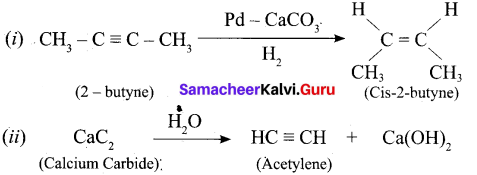Question 18.
How will you test sulfur present in organic compounds?
To a portion of the Lassaigne’s extract, freshly prepared sodium nitroprusside solution is added. If deep violet or purple colour is formed, the presence of sulfur is confirmed.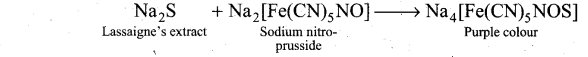Question 19.
Which one of the following has highest bond order? []\mathbf{N}_{2}, \mathbf{N}_{2}^{+} \text {or } \mathbf{N}_{2}^{-}[/latex]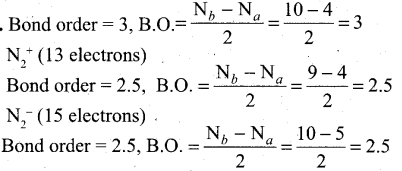So N2 has the highest bond order.

Question 20.
Define the term isotonic solution.

1. Two solutions having same osmotic pressure at a given temperature are called isotonic solutions.
2. When such solutions are separated by a semipermeable membrane, solvent flow between one to the other on either direction is same, i.e., the net solvent flow between two isotonic solutions is zero.Question 21.
A sample of gas has a volume of 8.5 dm3 at an unknown temperature. When the sample is submerged in ice water at 0°C, its volume gets reduced to 6.37 dm3. What is its initial temperature?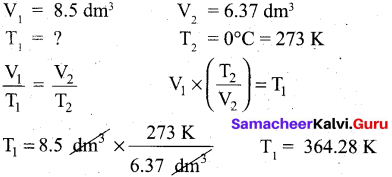Question 22.
Mention the uses of washing soda (or) sodium carbonate.

1. Sodium carbonate is highly used in laundering.
2. It is an important laboratory reagent used in the qualitative analysis and in volumetric analysis.
3. It is also used in water treatment to convert the hard water to soft water.
4. It is used in the manufacture of glass, paper and paint.

Question 23.
What is water – gas shift reaction?
The carbon monoxide of water gas can be converted to carbon dioxide by mixing the gas mixture with more steam at 400°C and passed over a shift converter containing iron/copper catalysts. This reaction is called water-gas shift reaction.
CO + H2O → CO2 + H2

Question 24.
What are Ψ and Ψ2?

1. Ψ itself has no physical meaning but it represents an atomic orbital.
2. Ψ2 is related to the probability of finding the electrons within a given volume of space.Part-III

Answer any six questions in which question No. 29 is compulsory. [16 x 3 = 18]

Question 25.
Distinguish between oxidation and reduction.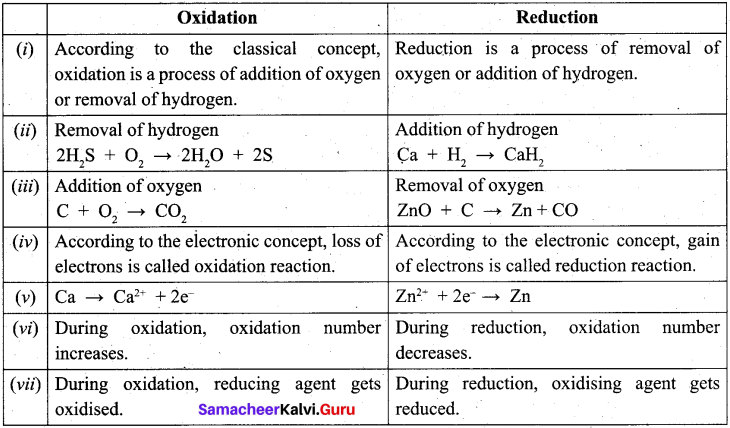Question 26.
Why there is a need for classification of elements?

1. Classification is a fundamental and essential process in our day-to-day life for the effective utilization of resources, daily events and materials.
2. In such a way, for the effective utilization of discovered elements becomes fundamentally essential process.
3. The periodic classification of the elements is one of the outstanding contributions to the progress of chemistry.Question 27.
Write the balanced equation for following reaction.
(i) Li metal with N2 gas
(ii) Heating solid sodium bicarbonate
(iii) Solid potassium hydroxide with CO2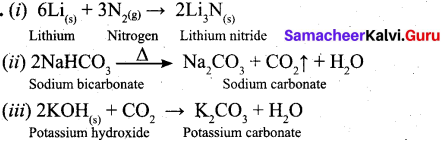Question 28.
Discuss briefly the similarities between Be and Al.

• Beryllium and aluminium have same electronegativity values.
• Their changes per unit area is closer.
• BeCl2 and AlCl3 forms dimeric structure. Both are soluble in organic solvents and are strong Lewis acids.
• Be(OH)2 and Al(OH)3 dissolves in excess alkali to give beryllate ion [Be(OH)4]3- and aluminate
• Be and A1 ions have strong tendency to form complexes, e.g. $$\mathrm{BeF}_{4}^{2}$$ and $$\mathrm{AlF}_{6}^{3-}$$.
• Both Be(OH)2 and Al(OH)3 are amphoteric in nature.
• Carbide of Be – beryllium carbide (Be2C) and A1 – aluminium carbide (Al4C3) give methane on hydrolysis.
• Both beryllium and aluminium are rendered passive by nitric acid.Question 29.
A tank contains a mixture of 52.5 g of oxygen and 65.1 g of CO2 at 300 K the total pressure in the tank is 9.21 atm. Calculate the partial pressure (in atm) of each gas in the mixture?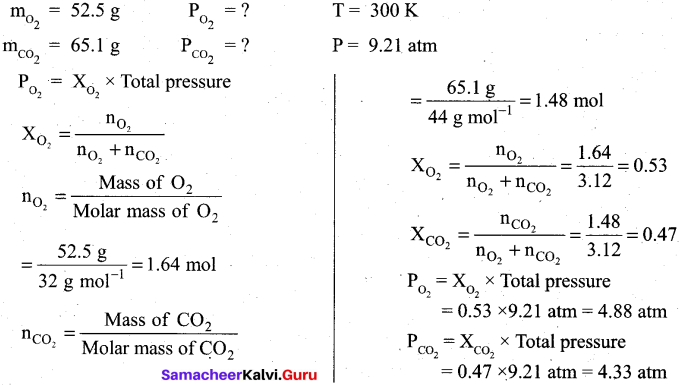Question 30.
Why He2 molecule does not exist?
Diatomic Helium molecule, He2 (Hypothetical). The electron configuration of Helium (Z = 2) in the ground state is Is2. As each Helium atom contains two electrons, there will be 4 electrons in He2 molecule. Keeping in view the Aufbau principle and Pauli’s exclusion principle its electron configuration would be as follows.
He2 : (σls)2ls)2.
The molecular orbital energy level diagram of He2: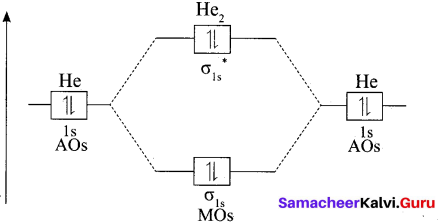Here, Nb = 2 and Na = 2
Bond order = $$\frac{N_{b}-N_{a}}{2}=\frac{2-2}{2}=0$$
As the bond order for He2 comes out to be zero, this molecule does not exist.Question 31.
Write all the possible isomers of molecular formula C4H10O and identify the isomerisms found in them.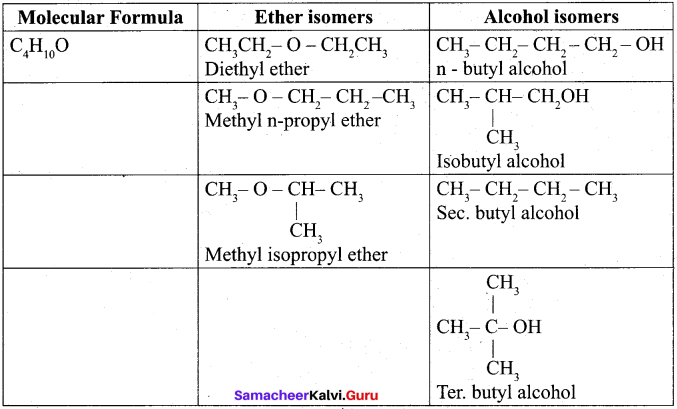Question 32.
Explain how oxygen deficiency is caused by carbon monoxide in our blood? Give its effect.

1. Carbon monoxide binds with haemoglobin and form carboxy the haemoglobin which impairs normal oxygen transport by blood and hence the oxygen carrying capacity of blood is reduced.
2. This oxygen deficiency results in headache, dizziness, tension, loss of consciousness, blurring of eyesight and cardiac arrest.Question 33.
Complete the reaction: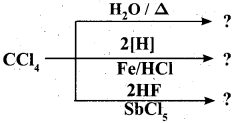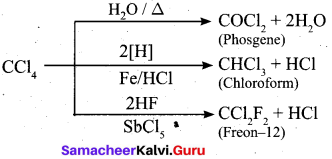Part – IV

Answer all the questions. [5 x 5 = 25]

Question 34.
(a) (i) How many orbitals are possible for n = 4? (2)
(ii) What are the limitations of Bohr’s model of an atom? (3)

[OR]

(b) (i) LiClO4 is more soluble than NaClO4. Why? (2)
(ii) Explain – IE1 of alkaline earth metals are higher than that of alkali metals. (3)
(a) (i) If n = 4, the possible number of orbitals are calculated as follows.
n = 4 – main shell = N
If n = 4 – l values are 0, 1, 2, 3
If l = 0 – 4s orbital = 1 orbital
If l = 1 – m = -l, 0, + 1 = 3 orbitals
If l = 2 – m = -2, -1, 0, + 1, + 2 = 5 orbitals
If l = 3 – m = -3, -2, -1, 0, +1, +2 , +3 = 7 orbit
∴ Total number of orbitals =16 orbitals(ii) Limitations of Bohr’s atom model:
1. The Bohr’s atom model is applicable only to species having one electron such as hydrogen, Li2+ etc and not applicable to multi-electron atoms.
2. It was unable to explain the splitting of spectral lines in the presence of magnetic field (Zeeman effect) or an electric field (Stark effect).
3. Bohr’s theory was unable to explain why the electron is restricted to revolve around the nucleus in a fixed orbit in which the angular momentum of the electron is equal to nh/2π.

[OR]

(b) (i) The small size of the Li+ ion means that it has a very high enthalpy of hydration and so lithium salts are much more soluble than the salts of other group 1. E.g. LiClO4 is upto 12 times more soluble than NaCIO4.

(ii)

• IE1 of alkaline earth metals > IE1 of alkali metals.
• IE2 of alkaline earth metals < IE2 of alkali metals.
• This occurs because in alkali metals the second electron is to be removed from a cation, which has already acquired a noble gas configuration.
• In the case of alkaline earth metals, the second electron is to be removed from a monovalent cation, which still has one electron in the outermost shell.
• Thus, the second electron can be removed more easily in the case of group 2 elements than in group 1 elements.Question 35.
(a)-(i) Can a Vanderwaal’s gas with a = 0 be liquefied? Explain. (2)
(ii) Deep sea divers ascend slowly and breath continuously by time they reach the surface. Give reason. (3)

[OR]

(b) (i) What are spontaneous reactions? (2)
(ii) Prove that for an ideal gas, Cp is greater than Cv (3)
(a) (i)

1. a = 0 for a Van der Waals gas i. e. for a real gas. Van der Waals constant a = 0. It cannot be liquefied.
2. If a = 0, there is a very less interaction between the molecules of gas.
3. ‘a’ is the measure of strength of Van der Waals force of attraction between the molecules of the gas. ,
4. If a is equal to zero, the Van der Waals force of attraction is very less and the gas cannot be liquefied.

(ii)

1. For every 10 m of depth, a diver experiences an additional 1 atm of pressure due to the weight of water surrounding him.
2. At 20 m, the diver experiences a total pressure of 3 atm. So the most important rule in diving is never hold breath.
3. Divers must ascend slowly and breath continuously allowing the regulator to bring the air pressure in their lungs to 1 atm by the time they reach the surface.[OR]

(b) (i) A reaction that does occur under the given set of conditions is called a spontaneous reaction. The expansion of a gas into a evacuated bulb is a spontaneous process, the reverse process that is gathering of all molecules into one bulb is not spontaneous. This example shows that processes that occur spontaneously in one direction cannot take place in opposite direction spontaneously.

Increase in randomness favours a spontaneous change. If enthalpy change of a process is negative, then the process is exothermic and occurs spontaneously. Therefore ΔH should be negative. If entropy change of a process is positive, then the process occurs spontaneously, therefore ΔS should be positive. If free energy of a process is negative, then the process occurs spontaneously, therefore AG should be negative.

For a spontaneous, irreversible process, ΔH < 0, ΔS > 0. ΔG < 0. i. e., ΔH = -ve, ΔS = +ve and ΔG = -ve.

(ii)

• It is clear that two heat capacities are not equal and Cp is greater than Cv by a factor which is related to the work done.
• At a constant pressure, a part of heat absorbed by the system is used up in increasing the internal energy of the system and the other for doing work by the system.
• At constant volume, the whole of heat absorbed is utilized in increasing the temperature of the system as there is no work done by the system.
Thus Cp is greater than Cv.
• By definition, H= U+ PV for I mole of an ideal gas.
H = U + RTBy differentiating this equation with respect to temperature T, we get, $$\frac{d H}{d T}=\frac{d U}{d T}+R$$
Cp = Cv + R; Cp – Cv = R

Thus for an ideal gas, Cp is greater than Cv by the gas constant R.

Question 36.
(a) (i) State law of mass action. (2)
(ii) Deduce the Vant IÌoff equation. (3)

[OR]

(b) (i) What is a π – bond? (2)
(ii) Discuss the formation of N2 molecule using MO theory. (3)
(a) (i) The law states that “At any instant, the rate of chemical reaction at a given temperature is directly proportional to the product of the active masses of the reactants at that instant.”
(ii) Vant hoff equation: This equation gives the quantitative temperature dependance of equilibrium constant K.

The relation between standard free energy change G° and equilibrium constant is
ΔG° = -RTlnK ……………………………… (1)
We know that, ΔG° = ΔH° — TΔS° ……………………………… (2)
Substituting (1) in equation (2)
– RTlnK = ΔH° – TΔS°
Rearranging, InK = $$\frac{-\Delta \mathrm{H}^{\circ}}{\mathrm{RT}}+\frac{\Delta \mathrm{S}^{\circ}}{\mathrm{R}}$$ ……………………………… (3)
Differentiating equation (3) with respect to temperature
$$\frac{d(\ln \mathrm{K})}{d \mathrm{T}}=\frac{\Delta \mathrm{H}^{\circ}}{\mathrm{RT}^{2}}$$ ……………………………… (4)Equation (4) is known as differential form of van’t Hoff equation. On integrating the equation 4, between T1 and T2 with their respective equilibrium constants K1 and K2,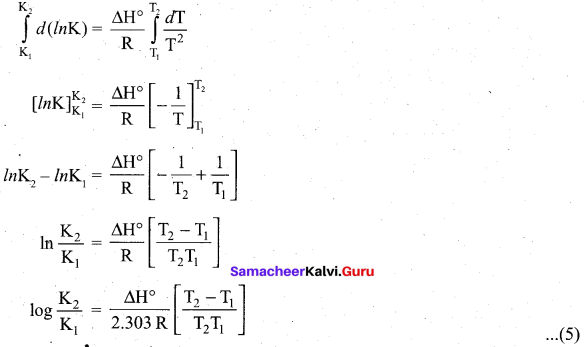Equation (5) is knowi as integrated fónn of van’t Hoff equation.

(b) (i) Pi-bond: When two atomic orbitals overlap sideways, the resultant covalent bond is called a pi (π) bond.
(ii) Formation of N, molecule using MO theory: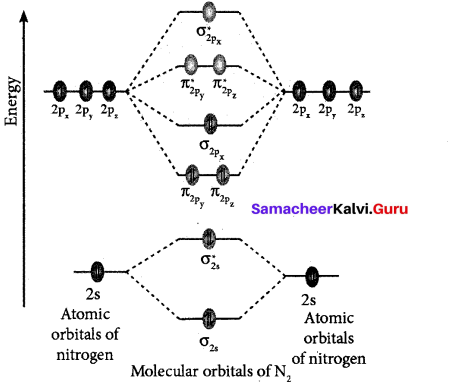1. Electronic configuration of N atom ls2 2s2 2p3.
2. Electronic configuration of N2 molecule is: $$\sigma 1 s^{2} \sigma^{*} 1 s^{2} \sigma 2 s^{2} \sigma^{*} 2 s^{2} \pi 2 p_{y}^{2} \pi 2 p_{z}^{2} \sigma 2 p_{x}^{2}$$
3. Bond order = $$\frac{N_{b}-N_{a}}{2} \frac{10-4}{2}=3$$
4. Molecule has no unpaired electrons hence, it is diamagnetic’.

Question 37.
(a) (i) Explain – Column chromatography. (3)
(ii) Give the structure for the following compound. (2)
(a) Tertiary butyl iodide
(b) 3 – Chlorobutanol

[OR]

(b)
(i) Explain – electromeric effect. (3)
(ii) Give the examples for β – elimination. (2)
(a) (i) Column chromatography:

1. The principle behind chromatography is selective distribution of mixture of organic substances between two phases a stationary phase and a moving phase. The stationary phase can be a solid or liquid, while the moving phase is a liquid or a gas. When the stationary phase.is a solid, the moving phase is a liquid or gas.
2. If the stationary phase is solid, the basis is adsorption, and when it is a liquid, the basis is partition.
3. Chromatography is defined as a technique for the separation of a mixture brought about by differential movement of the individual component through porous medium under the influence of moving solvent.
4. In column chromatography, the above principle is carried out in a long glass, column.

(ii) (a) Tertiary butyl iodide =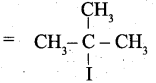(b) 3 – Chlorobutanol =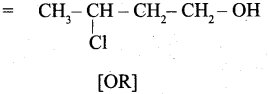[OR]

(b) (i) Electromeric effect.
1. The electromeric effect refers to the polarity produced in a multiple bonded compound when it is attacked by a reagent when a double or a triple bond is exposed to an attack by an electrophile the two n electrons which from the n bond are completely transferred to one atom or the other.

2. When a nucleophile approaches the carbonyl compound, the π -electrons between C and O is instantaneously shifted to the more electronegative oxygen. This make the carbon electron deficient and thus facilitating the formation of a new bond between the incoming nucleophile and the carbonyl carbon atom.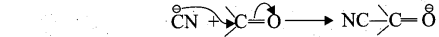3. When an electrophile such as H+ approaches an alkene molecule the n electrons are instantaneously shifted to the electrophile and a new bond is formed between carbon and hydrogen. This makes the other carbon electron deficient and hence it acquires a positive charge.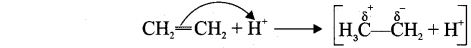4. This effect denotes as E-effect.
(ii) β-elimination: Elimination reactions involve the cleavage of a π bond and formation of a n bond. A nucleophilic pair of electrons heads into a new n bond as a leaving group departs. This process is called β-elimination because the bond P to the nucleophilic pair of electrons breaks.Example:
1. n-propyl bromide on reaction with alcoholic KOH give propene. In this reaction hydrogen and Br are eliminated.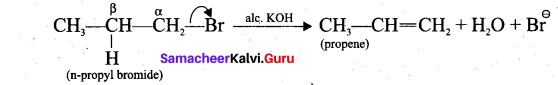2. Acid-catalysed dehydration of alcohols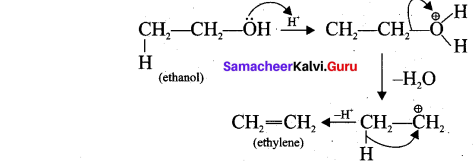Question 38.
(a) An organic compound A of a molecular formula C3H6, reacts with HBr to give B which is the major product for this reaction. A again reacts with HBr in presence of benzoyl peroxide to give C. Identify A, B and C. Explain the reactions. (5)

[OR]

(b) (i) Explain -The Bhopal Tragedy. (3)
(ii) What are the particulate that cause the pollutions in troposphere? (2)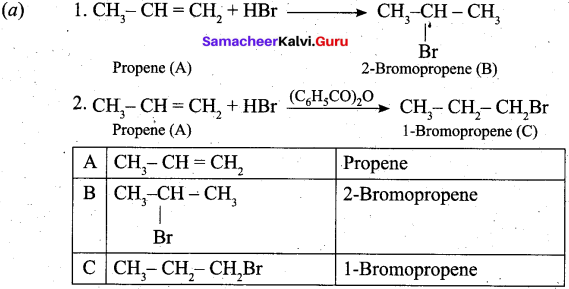[OR]

(b) (i) On 3rd Decembers, 1984 at Bhopal city in India, by the early morning, an explosion at Union Carbide pesticide plant released a cloud of toxic gas (Methyl isocyanate) CH3NCO into the air. Since the gas is twice as heavy as air, it did not drift away but formed a blanket over the surrounding area. It attacked people’s lungs and affect their breathing staying there or in the nearby areas. Thousands of people died and lives of many were ruined. The lungs, brain, eyes, muscles, as well as the gastrointestinal, neurological and immune systems of those people who survived, were severely affected.

(ii)
1. Viable particulates : The viable particulates are the same size, living organisms such as bacteria, fungi, moles, algae, etc. which are dispersed in air. Some of the fungi cause allergy in human beings and disease in plants.
2. Non-Viable particulates : The Non-viable particulates are small particles and liquid droplets suspended in air. They help in the transporting of particulates in the atmosphere.

They are classified according to

• smoke
• dust
• mists
• fumes.